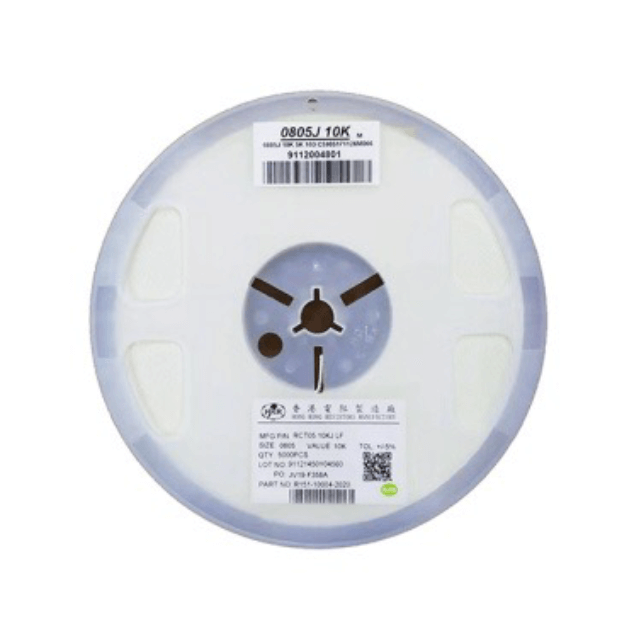English/ / / LED Chip ResistorShare to:

# LED Chip ResistorPacking：Tape on reel

Tolerance：±1%, ±5%

Resistance Value Range：1 OHM - 100 MEG OHM

HKR products comply with environmental requirement in accordance with SONY of code no. SS-00259 for not related in 8 harmful materials.

· Small size and ligtweight with size range per int'l standard

· Highly stable in auto-placement surface mounting application

· Compatible with both wave soldering and reflow soldering

Configuration

LED Chip Resistor

Dimensions

LED Chip Resistor

Power Derating Curve

LED Chip Resistor

LED Series

LED Chip Resistor

The working voltage is calculated based on the resistance value following the formula of V=√(P*R) or to its maximum extent as indicated above.

The overload voltage is calculated based on the resistance value following the formula of V=2.5√(P*R) or to i maximum extent as indicated above.

Soldering Temperature Curve

LED Chip Resistor

Specification And Test Methods

LED Chip Resistor

Markings

LED Chip Resistor

1.1 No markings on 0201 and 0402. Markings on the other sizes are expressed by a 3-digit code in its exact value

E. G. ： 6R8=6.8;100=10×10 0=10;472=47×102=4700=4.7K

1.2.+/-1% Resistance value markings

a. Markings on sizes 0805, 1206 and above are expressed by a 4-digit code.

E. G. ：82R5=82.5;1000=100×10 0=100;2212=221×102=22100=22.1K。

b. Markings on 0603 ±1%( IEC E–96 Series ) are expressed by a 3-digit code; the first two digits represent the value code and the last capital letter represents the multiplier.

The coding system of the E-96 series is as follows :

LED Chip Resistor

Multiplier code：

LED Chip Resistor

E. G. ：01Y=100× 10 -2=1;68A=499×100=499;02D=102×103=102K

c. Any resistance values of 1% tolerance but not included in the E-96 series, the value markings are the same as +/-5% tolerance with labelling identification.

d. Marking of the 0 Ohm resistor is a '0' with its value range from 0-50m?. No tolerance shown on the product label.

Tape And Reel Package

LED Chip Resistor

Outer Packaging

LED Chip Resistor

the first package：1~10 reels the second package：66 reels Max

· When quantity shall not reach the max, the remaining empty space shall be buried with buffer material.

· When the quantity is a few, altemative packing methods may be used. It is important to ensure the safety of the products during transportation.

LED Chip Resistor

Packing：Tape on reel

Tolerance：±1%, ±5%

Resistance Value Range：1 OHM - 100 MEG OHM

HKR products comply with environmental requirement in accordance with SONY of code no. SS-00259 for not related in 8 harmful materials.

· Small size and ligtweight with size range per int'l standard

· Highly stable in auto-placement surface mounting application

· Compatible with both wave soldering and reflow soldering

Configuration

LED Chip Resistor

Dimensions

LED Chip Resistor

Power Derating Curve

LED Chip Resistor

LED Series

LED Chip Resistor

The working voltage is calculated based on the resistance value following the formula of V=√(P*R) or to its maximum extent as indicated above.

The overload voltage is calculated based on the resistance value following the formula of V=2.5√(P*R) or to i maximum extent as indicated above.

Soldering Temperature Curve

LED Chip Resistor

Specification And Test Methods

LED Chip Resistor

Markings

LED Chip Resistor

1.1 No markings on 0201 and 0402. Markings on the other sizes are expressed by a 3-digit code in its exact value

E. G. ： 6R8=6.8;100=10×10 0=10;472=47×102=4700=4.7K

1.2.+/-1% Resistance value markings

a. Markings on sizes 0805, 1206 and above are expressed by a 4-digit code.

E. G. ：82R5=82.5;1000=100×10 0=100;2212=221×102=22100=22.1K。

b. Markings on 0603 ±1%( IEC E–96 Series ) are expressed by a 3-digit code; the first two digits represent the value code and the last capital letter represents the multiplier.

The coding system of the E-96 series is as follows :

LED Chip Resistor

Multiplier code：

LED Chip Resistor

E. G. ：01Y=100× 10 -2=1;68A=499×100=499;02D=102×103=102K

c. Any resistance values of 1% tolerance but not included in the E-96 series, the value markings are the same as +/-5% tolerance with labelling identification.

d. Marking of the 0 Ohm resistor is a '0' with its value range from 0-50m?. No tolerance shown on the product label.

Tape And Reel Package

LED Chip Resistor

Outer Packaging

LED Chip Resistor

the first package：1~10 reels the second package：66 reels Max

· When quantity shall not reach the max, the remaining empty space shall be buried with buffer material.

· When the quantity is a few, altemative packing methods may be used. It is important to ensure the safety of the products during transportation.

LED Chip Resistor

Previous:
Next:
Hong Kong Resistors Manufactory
Resistor Is Our Name